# 5. Let u = [0,1,1), v= (-5, -4,6.7], and P = (4, -5,6). In the following,...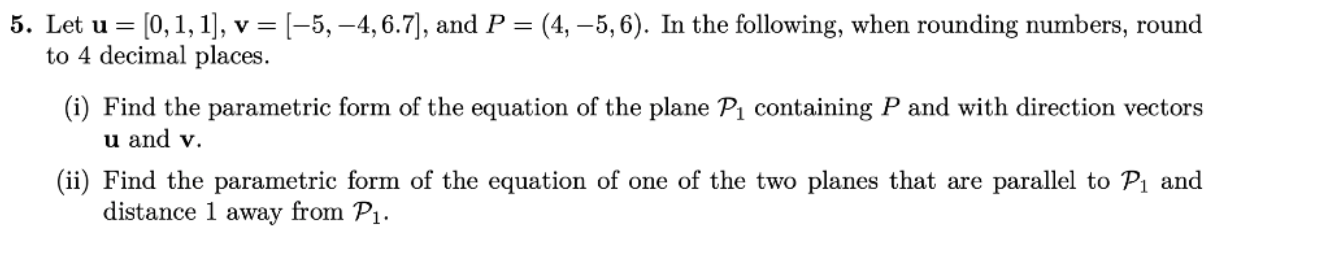5. Let u = [0,1,1), v= (-5, -4,6.7], and P = (4, -5,6). In the following, when rounding numbers, round to 4 decimal places. (i) Find the parametric form of the equation of the plane Pi containing P and with direction vectors u and v. (ii) Find the parametric form of the equation of one of the two planes that are parallel to P1 and distance 1 away from P1.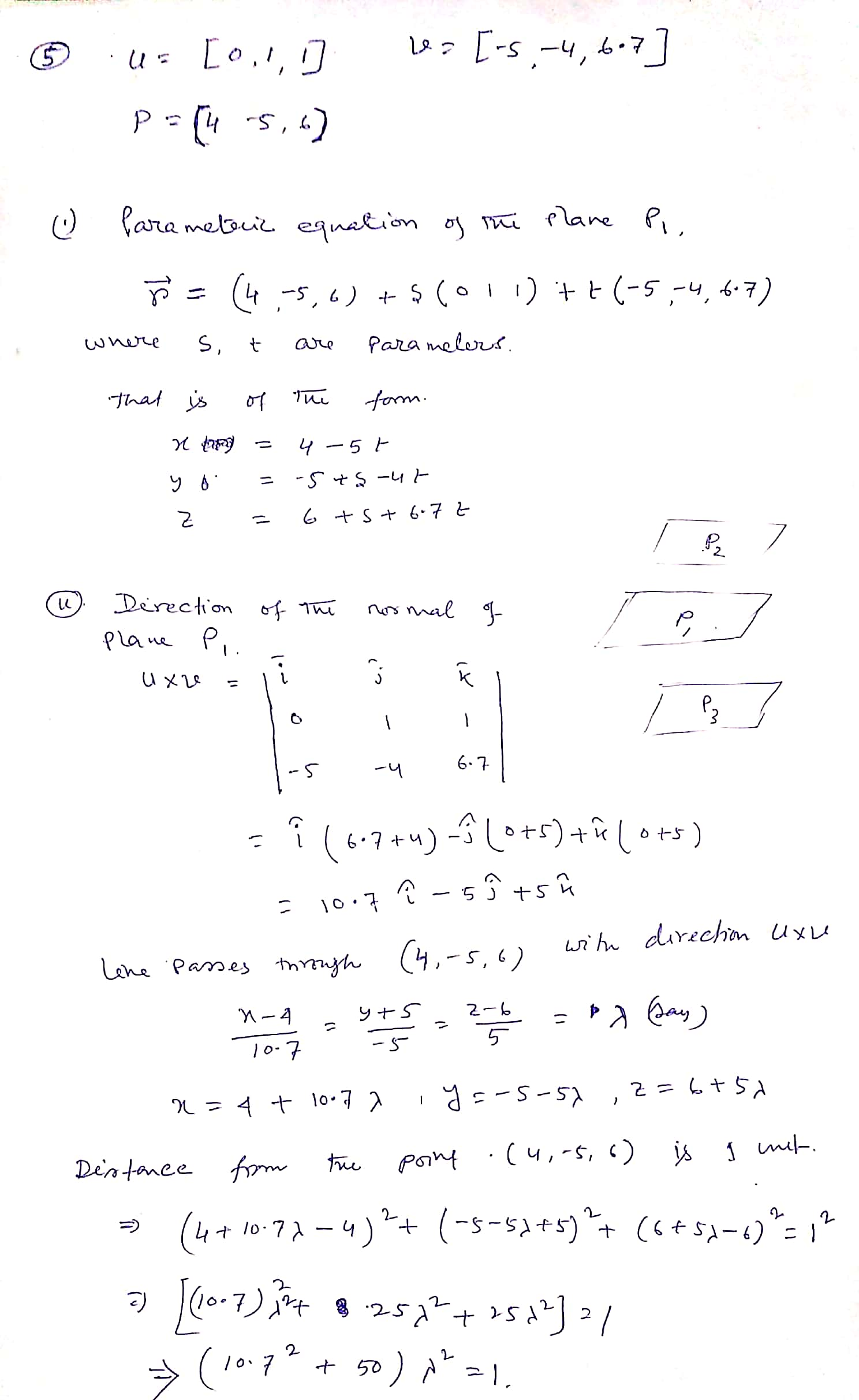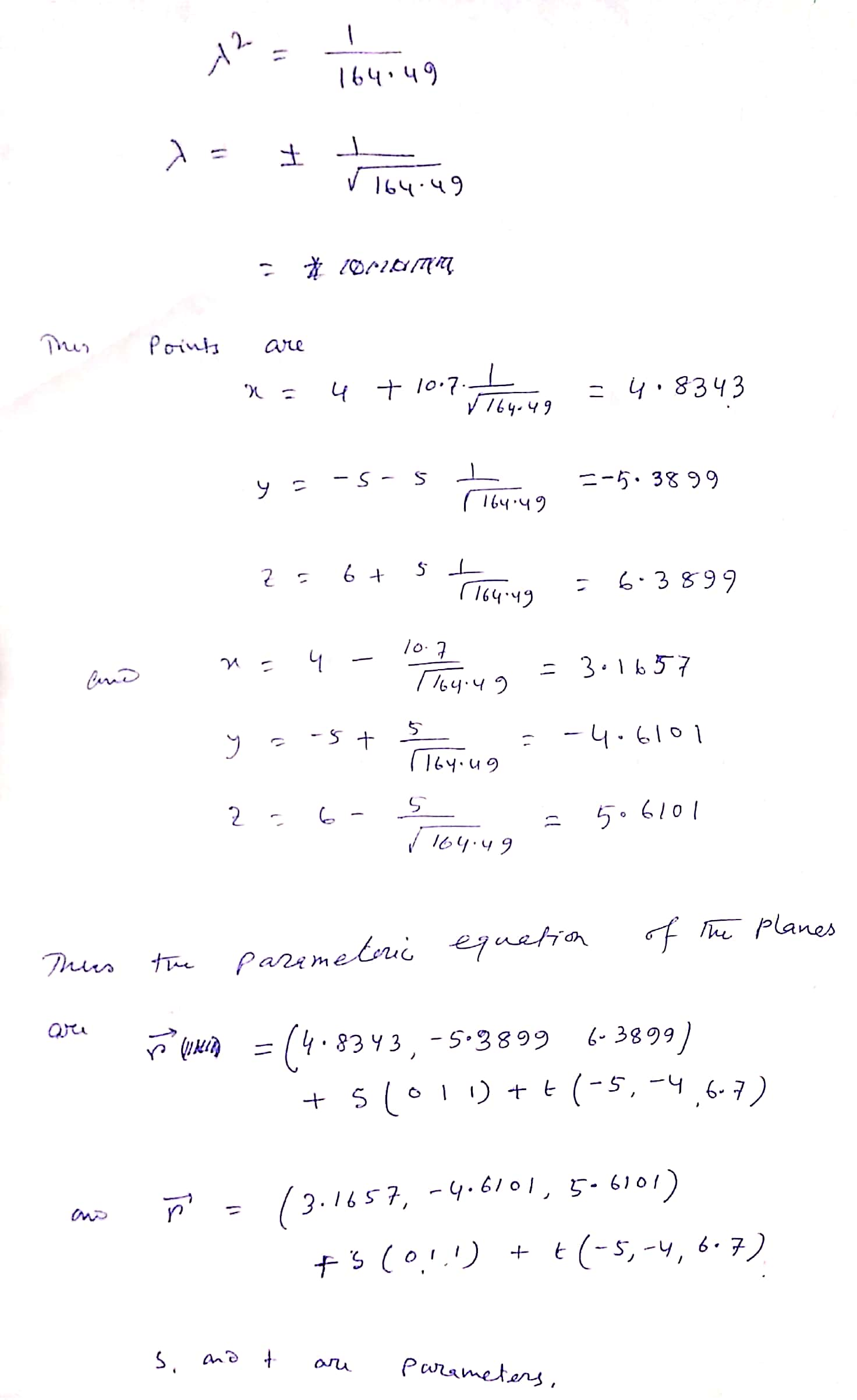##### Add Answer of: 5. Let u = [0,1,1), v= (-5, -4,6.7], and P = (4, -5,6). In the following,...
Similar Homework Help Questions
• ### 5. Let u = [0,1,1), v = (-5, -4,6.7), and P = (4.–5.6). In the following,...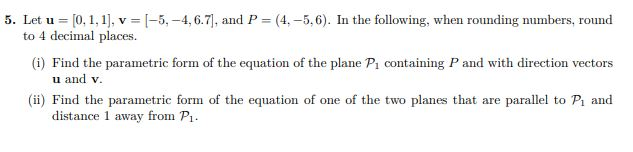5. Let u = [0,1,1), v = (-5, -4,6.7), and P = (4.–5.6). In the following, when rounding numbers, round to 4 decimal places. (i) Find the parametric form of the equation of the plane P. containing P and with direction vectors u and v. (ii) Find the parametric form of the equation of one of the two planes that are parallel to P, and distance 1 away from P1.

• ### 3. Consider the following system of linear equations: 2.0 + 2y + 2kz = 2 kx...3. Consider the following system of linear equations: 2.0 + 2y + 2kz = 2 kx + ky+z=1 2x + 3y + 72 = 4 (i) Turn the system into row echelon form. (ii) Determine which values of k give (i) a unique solution (ii) infinitely many solutions and (iii) no solutions. Show your working. 4. Solve the following system of linear equations using Gauss-Jordan elimination: x1 + x2 - 2.13 + 24 +3.25 = 1 2.x1 - x2 +...

• ### 1. Let Q = (-3.-3.-3.3), R = (-3.-3,-33) and S = (1,10,10.1). In the following, when...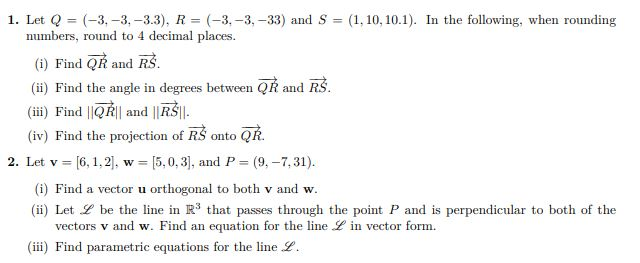1. Let Q = (-3.-3.-3.3), R = (-3.-3,-33) and S = (1,10,10.1). In the following, when rounding numbers, round to 4 decimal places. (i) Find QR and RS. (ii) Find the angle in degrees between QR and RS. (iii) Find ||QŘ|| and ||RŠI. (iv) Find the projection of R\$ onto QR. 2. Let v = [6, 1, 2], w = [5,0,3), and P = (9,-7,31). (i) Find a vector u orthogonal to both v and w. (ii) Let L be...

• ### 2. Let v= [6, 1, 2], w = [5,0, 3), and P= (9, -7,31). (i) Find...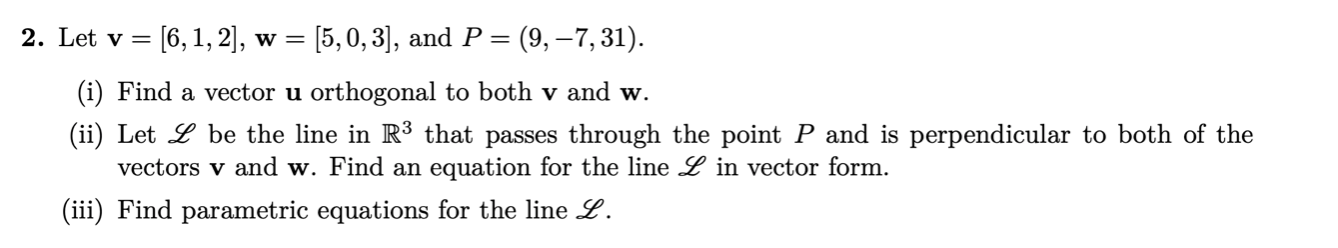2. Let v= [6, 1, 2], w = [5,0, 3), and P= (9, -7,31). (i) Find a vector u orthogonal to both v and w. (ii) Let L be the line in R3 that passes through the point P and is perpendicular to both of the vectors v and w. Find an equation for the line L in vector form. (iii) Find parametric equations for the line L.

• ### 1. Let Q = (-3, -3, -3.3), R = (-3, -3, -33) and S = (1,...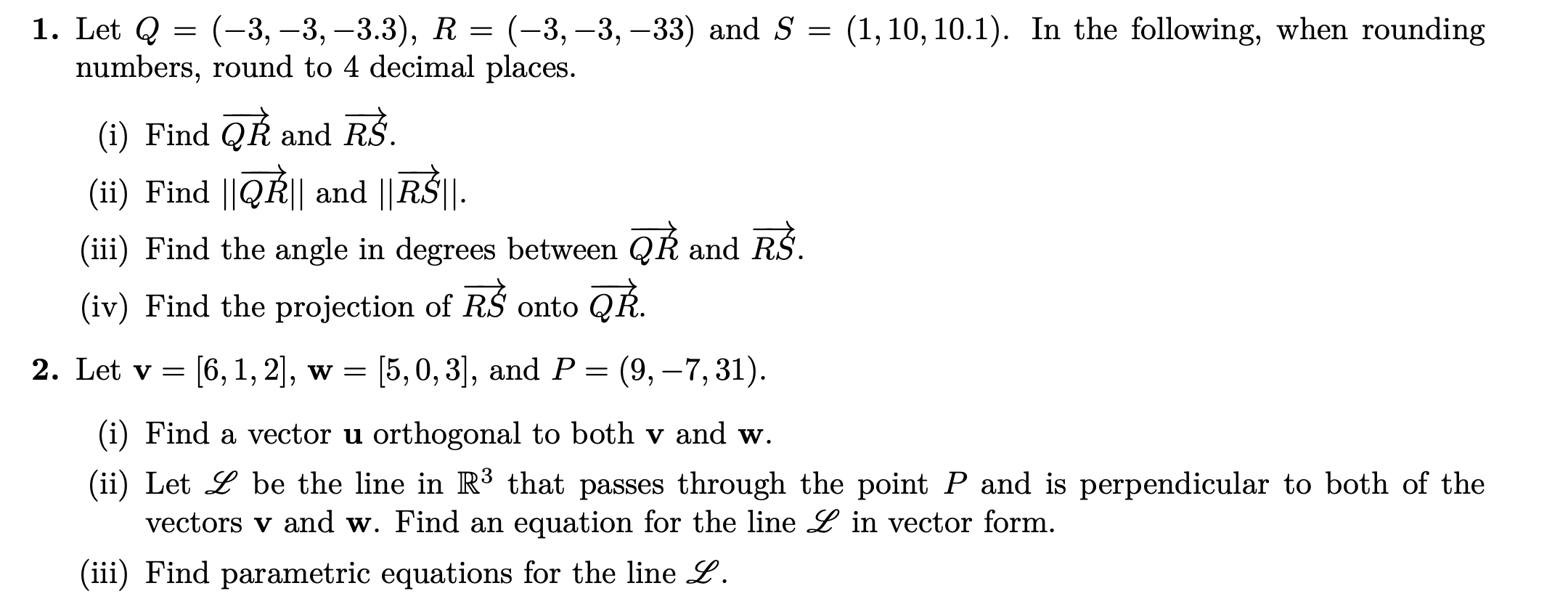1. Let Q = (-3, -3, -3.3), R = (-3, -3, -33) and S = (1, 10, 10.1). In the following, when rounding numbers, round to 4 decimal places. (i) Find QŘ and RS. (ii) Find ||QR|| and ||RŠI. (iii) Find the angle in degrees between QR and RS. (iv) Find the projection of RŠ onto QŘ. 2. Let v= [6, 1, 2], w = [5,0,3], and P = (9, -7,31). (i) Find a vector u orthogonal to both v...

• ### Problem l: Let u, v and w be three vectors in R3 (a) Prove that wlv +lvlw bisects the angle betwe...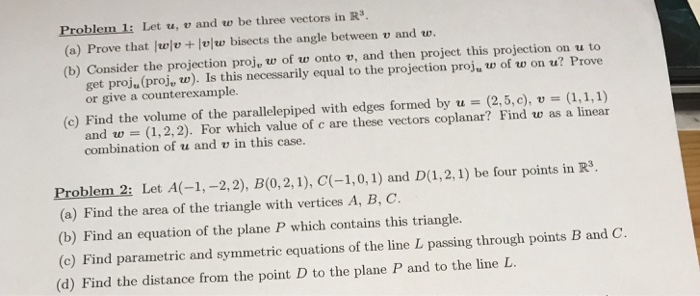Problem l: Let u, v and w be three vectors in R3 (a) Prove that wlv +lvlw bisects the angle between v and w. (b) Consider the projection proj, w of w onto v, and then project this projection on u to get proju (proj, w). Is this necessarily equal to the projection proj, w of w on u? Prove or give a counterexample. (c) Find the volume of the parallelepiped with edges formed by u-(2,5,c), v (1,1,1) and w...

• ### Vectors : If u and v are vectors such that..

|u|=2 , |v| = 3 and the angle between u and v is pi/3a)Find the exact value for |u - 2v|b) let u = <4,-8,1> , v = <2,1,-2> and w = <3,-4,12> determine if the vectors u,v and w are coplanar. Justify your answerc) Let u,v and w be vectors such that u . (v x w) = 3 Find(i) u.(w x v) (ii) (u x w).v (iii) v.(w x w)

• ### (a). Find the equation of the plane through Po = (1,2,1) with normal vector i =...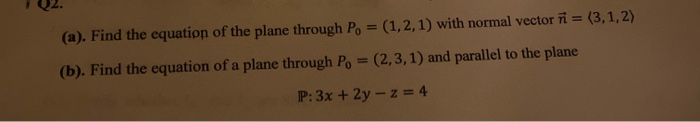(a). Find the equation of the plane through Po = (1,2,1) with normal vector i = (3,1,2) (b). Find the equation of a plane through Po = (2,3,1) and parallel to the plane P:3x + 2y -- z = 4 | Q4. Consider the line z-3 y-2 3 L, : * - - - L2: **** 2+5 y-3 -1 2 (i). Write the equations of both lines in parametric form (ii). Find the direction vectors V1, V2 of the lines...

• ### Let u =-3ǐ一4] and v-2i-6j. Decompose u into two vectors ui and u 2, where ui...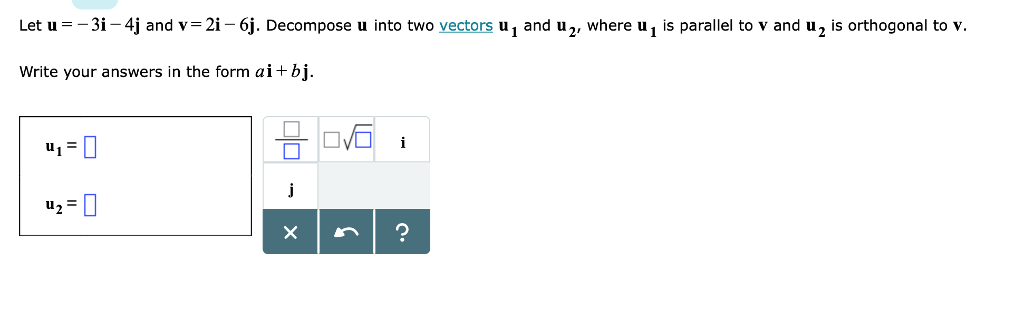Let u =-3ǐ一4] and v-2i-6j. Decompose u into two vectors ui and u 2, where ui is parallel to v and u 2 is orthogonal to v. Write your answers in the form ai+bj. u2

• ### Q2. Let u and v be non-parallel vectors in Rn and define Suv (a) Does the point r lie on the straight line through q with direction vector p? (b) Does the point s lie on the straight line through...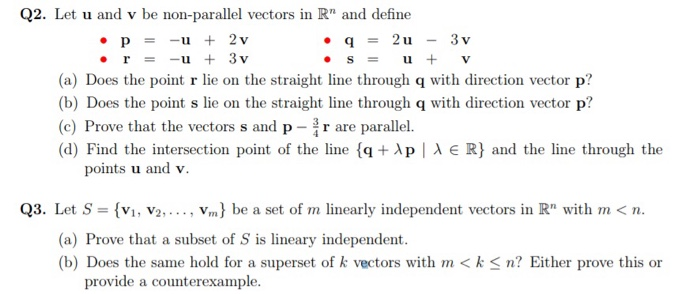Q2. Let u and v be non-parallel vectors in Rn and define Suv (a) Does the point r lie on the straight line through q with direction vector p? (b) Does the point s lie on the straight line through q with direction vector p? (c) Prove that the vectors s and p -r are parallel. (d) Find the intersection point of the line {q+λ p | λ E R} and the line through the points u and v. Q3....

Free Homework App